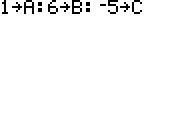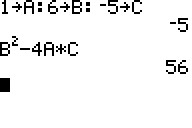# Knowledge Base

## Solution 28170: Finding the Discriminant of a Quadratic Equation Using the TI-84 Plus Family or TI-Nspire in TI-84 Plus Mode Handhelds.

### How can I find the Discriminant of a Quadratic equation on my TI-84 Plus family calculator or TI-Nspire in TI-84 Plus mode?

The TI-83 plus and TI-84 plus family do not have the built function to find discriminant. However, the instructions below show how to compute the discriminant by using variables:

The discriminant is a number that can be calculated from any quadratic equation. A quadratic equation is an equation that can be written as ax2 + bx + c = 0

The discriminant in a quadratic equation is found by the following formula and the discriminant provides critical information regarding the nature of the roots/solutions of any quadratic equation. discriminant= b-4ac
Critical information on the solutions:

• When b2-4ac = 0 then there are 2 equal roots.
• When b2-4ac > 0 then there are 2 different roots.
• When b2-4ac < 0 then there are no roots at all.

Example: Find the discriminant of the equation x2+6x-5=0

1) Press 1[STO][ALPHA][A][ALPHA][:]6[STO][ALPHA][B][ALPHA][:]-5[STO][ALPHA][C]
2) Press [ENTER]3) Type B2-4A*C
4) Press [ENTER] to display the discriminant. For this equation, the discriminant is 56.